• 俗话说流程决定绩效，而这一款整理发布的优化流程原则定能给你最好的流程参考，欢迎大家下载优...该文档为优化流程原则，是一份很不错的参考资料，具有较高参考价值，感兴趣的可以下载看看
• 易语言优化程序.rar 易语言优化程序.rar 易语言优化程序.rar 易语言优化程序.rar 易语言优化程序.rar 易语言优化程序.rar
• 易语言源码优化程序.rar 易语言源码优化程序.rar 易语言源码优化程序.rar 易语言源码优化程序.rar 易语言源码优化程序.rar 易语言源码优化程序.rar
• 原本打算好好写个线材优化程序，由于没有找到好的优化算法，写出来就成了这个东西了。其中优化结果是否最优存在问题；优化出来的结果也没进一步整理;其他一些辅助功能也没有加。呵呵，烂就烂吧，怎么说也是我第一次...
• 深度系统做的优化程序 非常好用，可以把内存占用优化到非常小，估计几十m的样子就可以了，大家可以试试看
• 关于wcdma优化流程的文档，对优化软件有一定的描述。wcdma
• pso 优化程序，主要是用在优化中的问题，程序不难!
• 基于遗传算法的电力系统无功优化程序，可以帮您更好的进行电力系统的优化，使用标准的MATLAB程序 基于遗传算法的电力系统无功优化程序，可以帮您更好的进行电力系统的优化，使用标准的MATLAB程序
• xp终极后台优化程序 终极优化xp终极后台优化程序 终极优化xp终极后台优化程序 终极优化xp终极后台优化程序 终极优化xp终极后台优化程序 终极优化xp终极后台优化程序 终极优化xp终极后台优化程序 终极优化
• 如何定位并优化慢查询SQL？如何使用慢查询日志？本文带来慢查询例子演示，新手都能看懂！那查询语句慢怎么办？explain带你分析sql执行计划！当主键索引、唯一索引、普通索引都存在，查询优化器如何选择？本文带你一...

继前篇博文看了这么多，终于理解了MySQL索引之后，给大家再来一篇日常开发需要用到的优化技巧。

文章目录
1.如何定位并优化慢查询SQL？2.如何使用慢查询日志？3.慢查询例子演示，新手都能看懂4.查询语句慢怎么办？explain带你分析sql执行计划5.当主键索引、唯一索引、普通索引都存在，查询优化器如何选择？

1.如何定位并优化慢查询SQL？
一般有3个思考方向 1.根据慢日志定位慢查询sql 2.使用explain等工具分析sql执行计划 3.修改sql或者尽量让sql走索引
2.如何使用慢查询日志？
先给出步骤，后面说明 有3个步骤 1.开启慢查询日志
首先开启慢查询日志，由参数slow_query_log决定是否开启，在MySQL命令行下输入下面的命令：
set global slow_query_log=on;

默认环境下，慢查询日志是关闭的，所以这里开启。
2.设置慢查询阈值
set global long_query_time=1;

只要你的SQL实际执行时间超过了这个阈值，就会被记录到慢查询日志里面。这个阈值默认是10s，线上业务一般建议把long_query_time设置为1s，如果某个业务的MySQL要求比较高的QPS，可设置慢查询为0.1s。发现慢查询及时优化或者提醒开发改写。一般测试环境建议long_query_time设置的阀值比生产环境的小，比如生产环境是1s，则测试环境建议配置成0.5s。便于在测试环境及时发现一些效率的SQL。
甚至某些重要业务测试环境long_query_time可以设置为0，以便记录所有语句。并留意慢查询日志的输出，上线前的功能测试完成后，分析慢查询日志每类语句的输出，重点关注Rows_examined（语句执行期间从存储引擎读取的行数），提前优化。
3.确定慢查询日志的文件名和路径
show global variables like 'slow_query_log_file'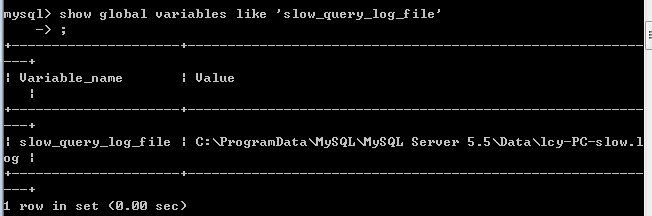结果会发现慢日志默认路径就是MySQL的数据目录，我们可以来看一下MySQL数据目录
 show global variables like 'datadir';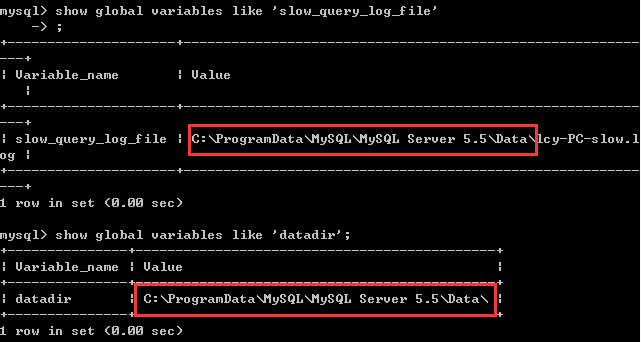不用关注这里为什么不是MySQL 8.0，这和版本没什么关系的。
来，直接上菜，干巴巴的定义我自己都看不下去
我们先来查看一下变量，我框出了需要注意的点 查询带有quer的相关变量
show global variables like '%quer%';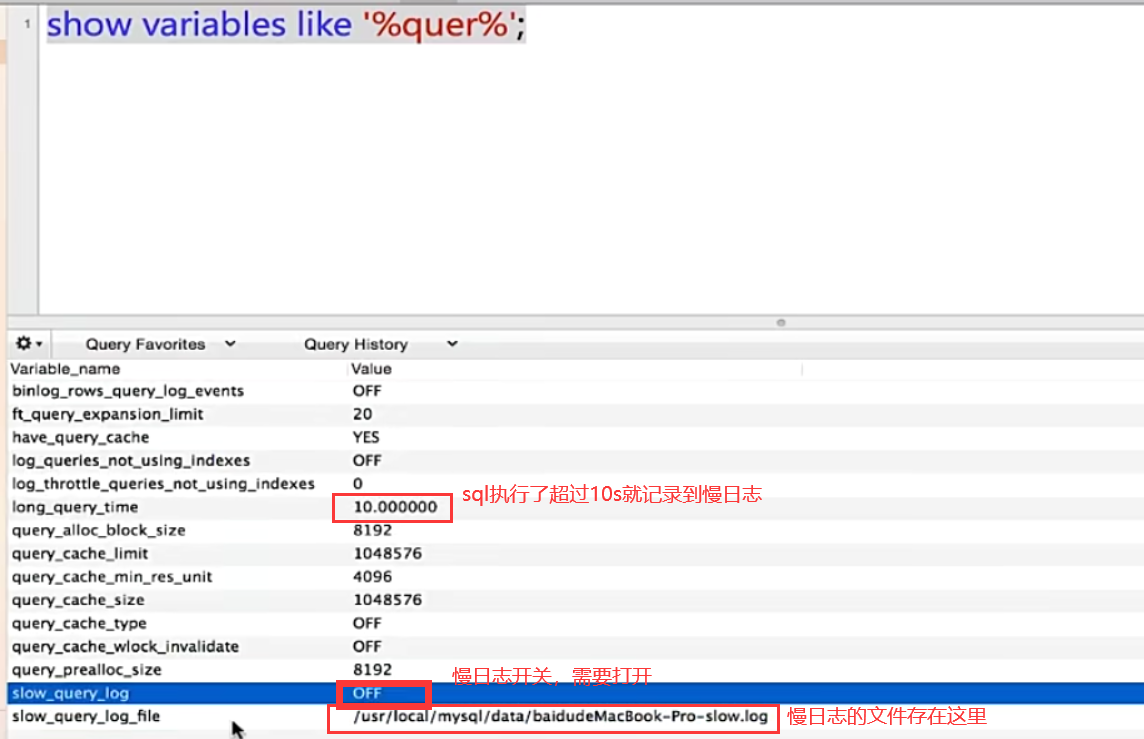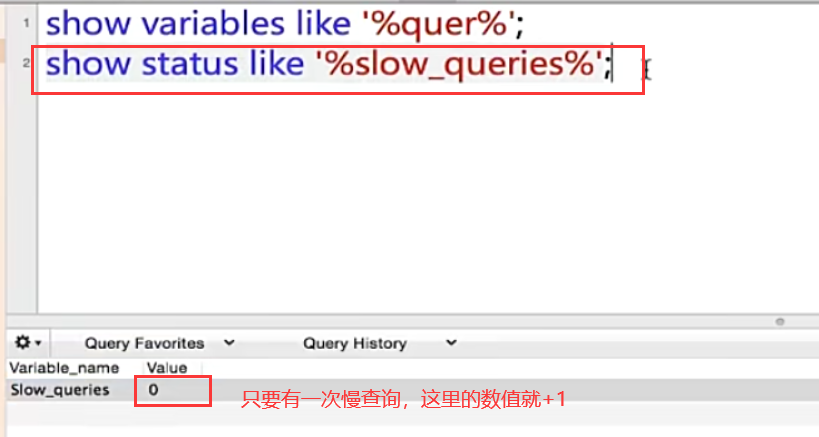这里设置慢查询阈值为1s
set global long_query_time=1;

可以看到已经修改过来了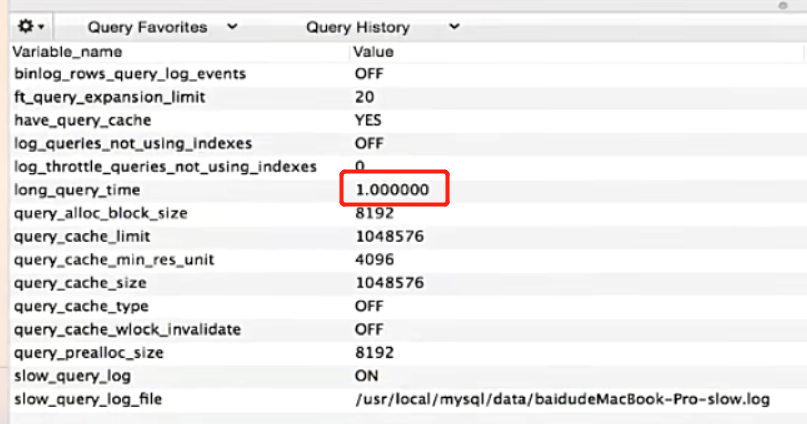但是重启mysql客户端设置和统计慢查询日志条数就会清零，即所有配置修改会还原
命令修改配置之后，在命令行net stop mysql关闭MySQL服务，再net start mysql开启MySQL服务，接着执行show global variables like '%quer%';会发现配置还原了。
在配置文件修改才能永久改变，否则重启数据库就还原了
3.慢查询例子演示，新手都能看懂
数据表结构，偷懒没写comment
CREATE TABLE person_info_large (
id BIGINT UNSIGNED NOT NULL AUTO_INCREMENT,
account VARCHAR (10),
name VARCHAR (20),
area VARCHAR (20),
title VARCHAR (20),
motto VARCHAR (50),
PRIMARY KEY (id),
UNIQUE(account),
KEY index_area_title(area,title)
) ENGINE = INNODB AUTO_INCREMENT = 1 DEFAULT CHARSET = utf8

这里的数据是200W条。请注意表结构，记住哪几个字段有索引即可，后续围绕这个表进行分析。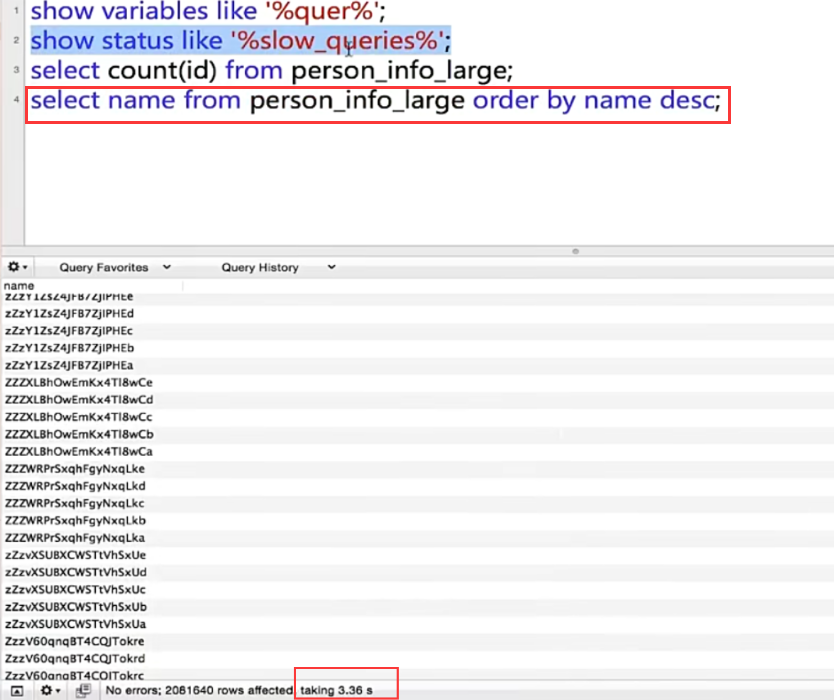这个3.36s并不是实际执行时间，实际执行时间得去慢查询日志去看Query_time参数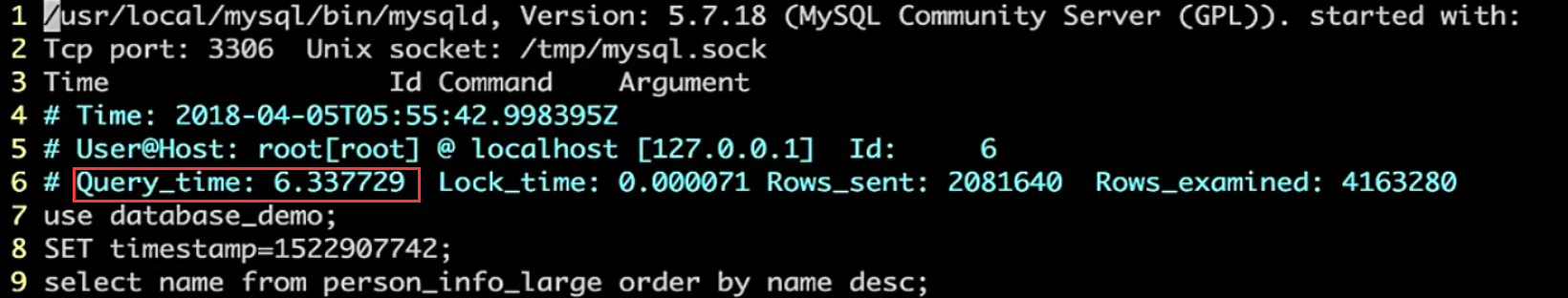可以看到Query_time: 6.337729s，超过了1s，所以会被记录，一个select语句查询这么久，简直无法忍受。

图中其他的参数解释如下： Time：慢查询发生的时间 Query_time：查询时间 Lock_time：等待锁表的时间 Rows_sent：语句返回的行数 Rows_exanined：语句执行期间从存储引擎读取的行数

上面这种方式是用系统自带的慢查询日志查看的，如果觉得系统自带的慢查询日志不方便查看，可以使用pt-query-digest或者mysqldumpslow等工具对慢查询日志进行分析。
注意：有的慢查询正在执行，结果已经导致数据库负载过高，而由于慢查询还没执行完，因此慢查询日志看不到任何语句，此时可以使用show processlist命令查看正在执行的慢查询。show processlist显示哪些线程正在运行，如果有PROCESS权限，则可以看到所有线程。否则，只能看到当前会话线程。
4.查询语句慢怎么办？explain带你分析sql执行计划
根据上一节的表结构可以知道，account是添加了唯一索引的字段。explain分析一下执行计划。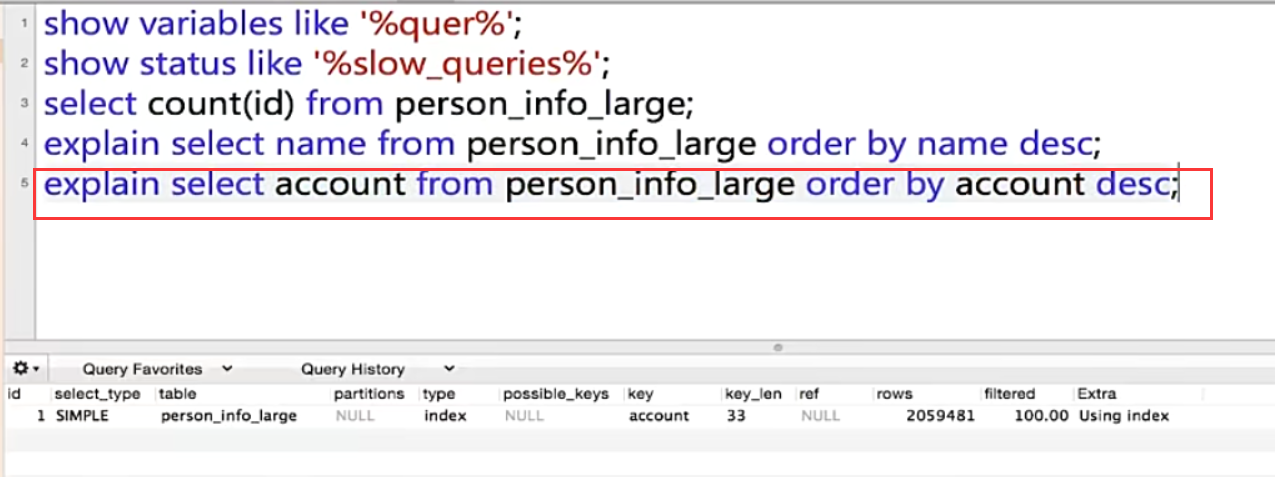我们重点需要关注select_type、type、possible_keys、key、Extra这些列，我们来一一说明，看到select_type列，这里是SIMPLE简单查询，其他值下面给大家列出。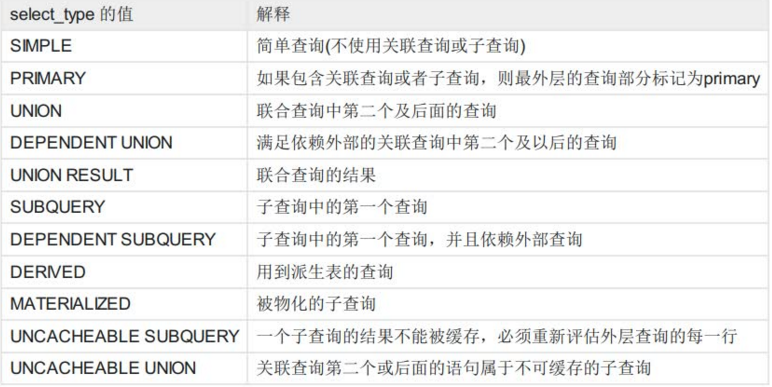type列，这里是index，表示全索引扫描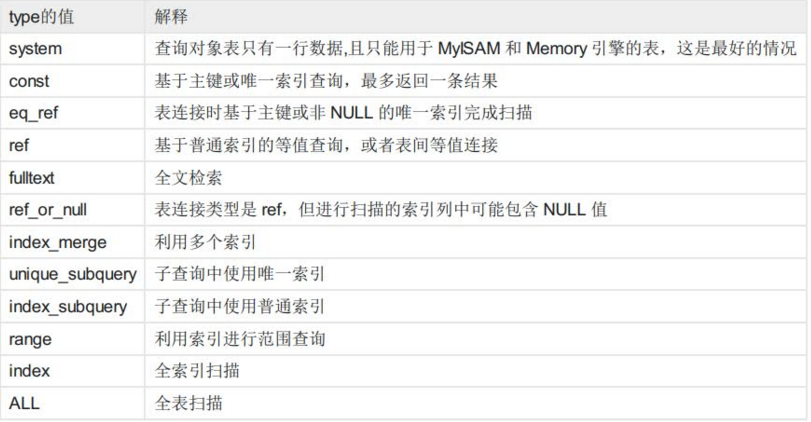表格从上到下代表了sql查询性能从最优到最差，如果是type类型是all，说明sql语句需要优化。

注意：如果type = NULL，则表明个MySQL不用访问表或者索引，直接就能得到结果，比如explain select sum(1+2);

possible_keys代表可能用到的索引列，key表示实际用到的索引列，以实际用到的索引列为准，这是查询优化器优化过后选择的，然后我们也可以根据实际情况强制使用我们自己的索引列来查询。
Extra列，这里是Using index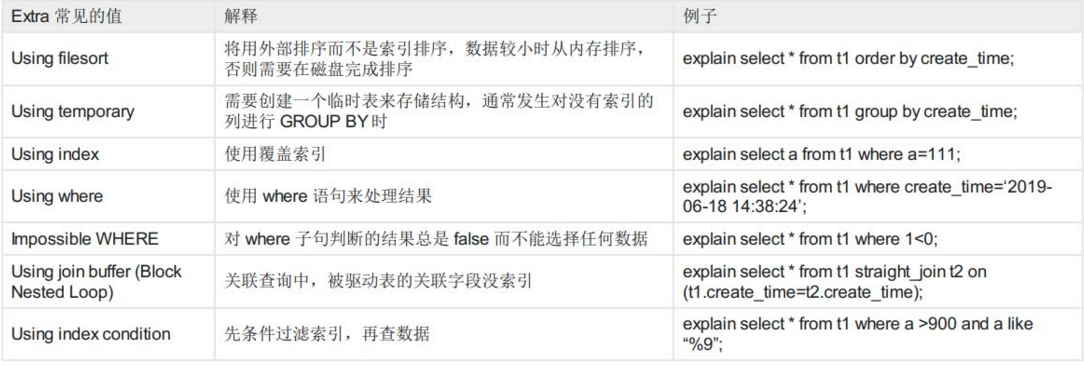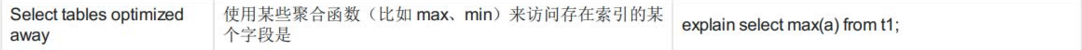一定要注意，Extra中出现Using filesort、Using temporary代表MySQL根本不能使用索引，效率会受到严重影响，应当尽可能的去优化。
出现Using filesort说明MySQL对结果使用一个外部索引排序，而不是从表里按索引次序读到相关内容，有索引就维护了B+树，数据本来就已经排好序了，这说明根本没有用到索引，而是数据读完之后再排序，可能在内存或者磁盘上排序。也有人将MySQL中无法利用索引的排序操作称为“文件排序”。
出现Using temporary表示MySQL在对查询结果排序时使用临时表，常见于order by和分组查询group by
回到上一个话题，我们看到account是添加了唯一索引的字段。explain分析了执行计划后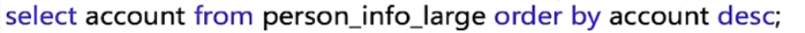直接按照account降序来查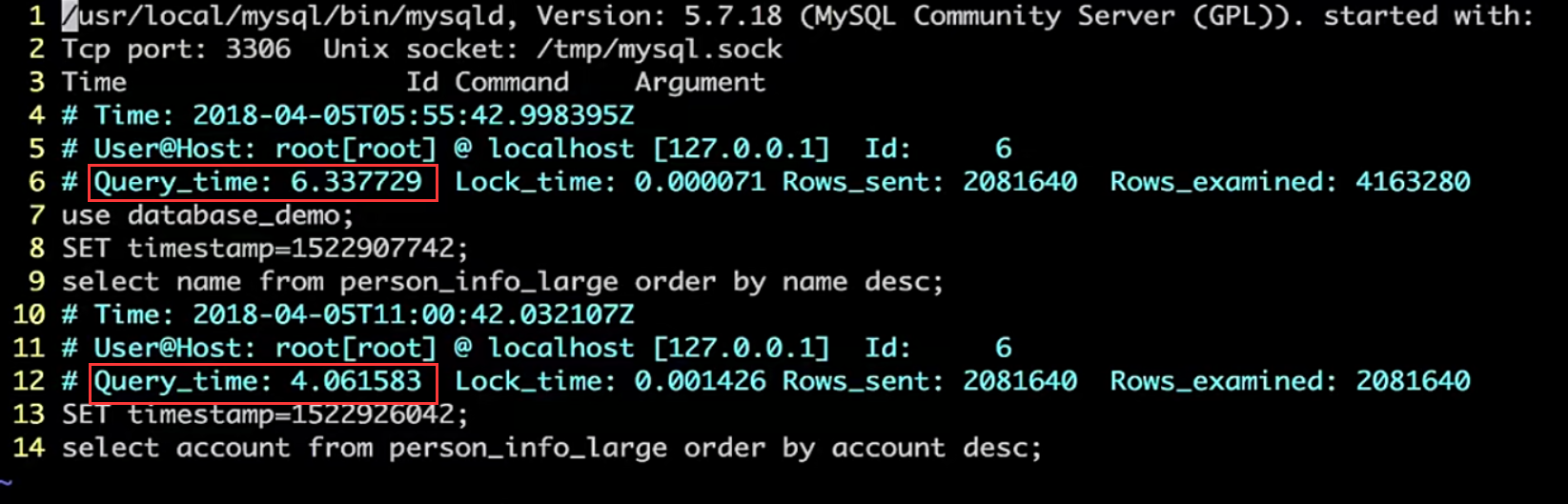查看慢查询日志发现，使用索引之后，查询200W条数据的速度快了2s
接着我们分析一下查询name的sql执行计划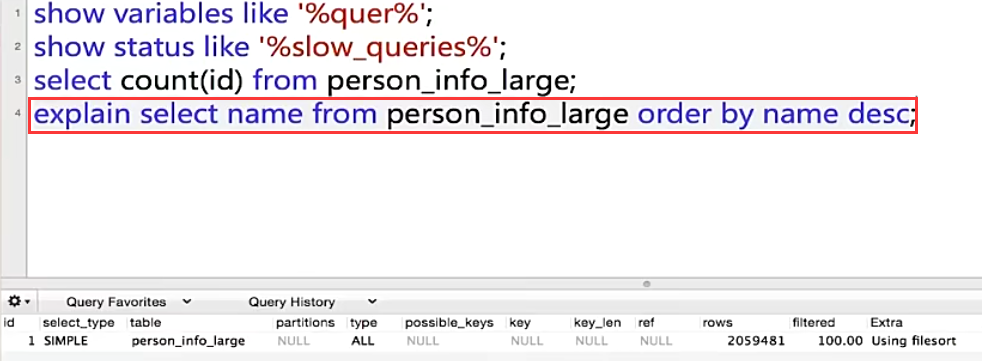然后给name字段加上索引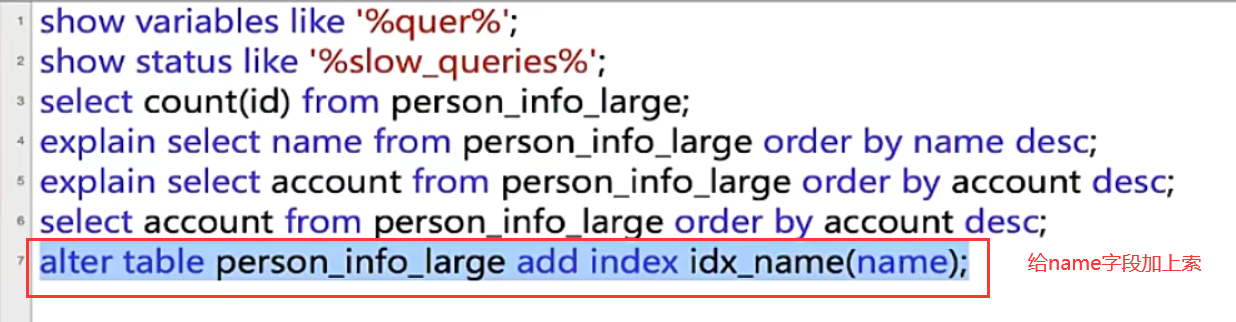加上索引之后，继续看看查询name的sql执行计划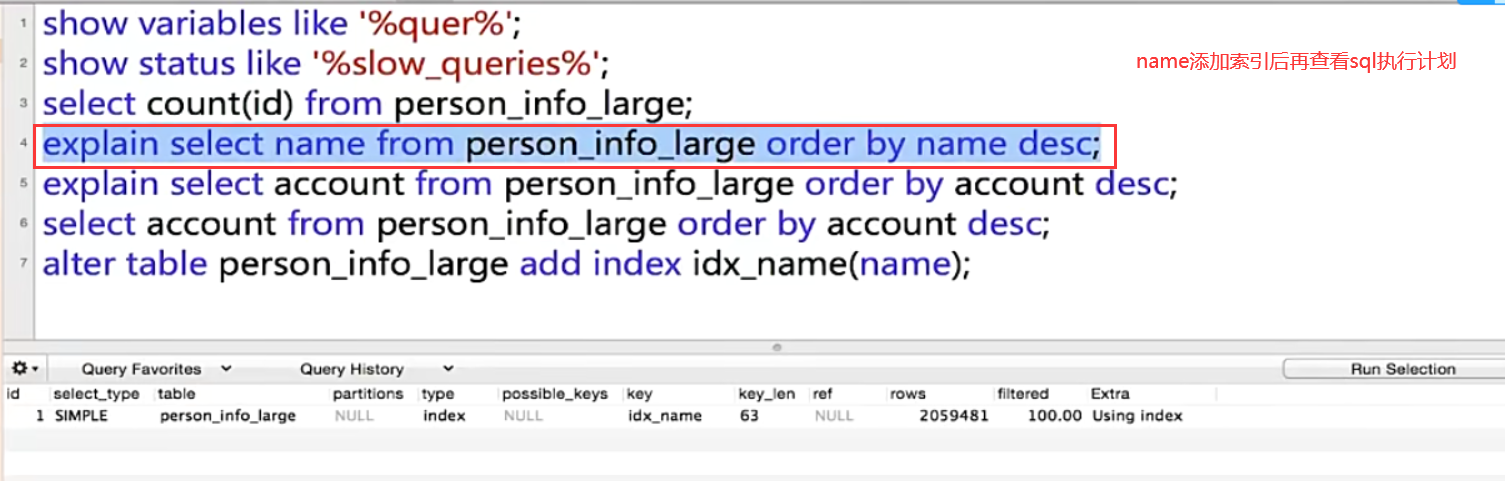对比一下前面name不加索引时的执行计划就会发现，加了索引后，type由ALL全表扫描变成index索引扫描。order by并没有 using filesort，而是using index，这里B+树已经将这个非聚集索引的索引字段的值排好序了，而不是等到查询的时候再去排序。
接着我们继续执行查询语句，此时name已经是添加了索引的。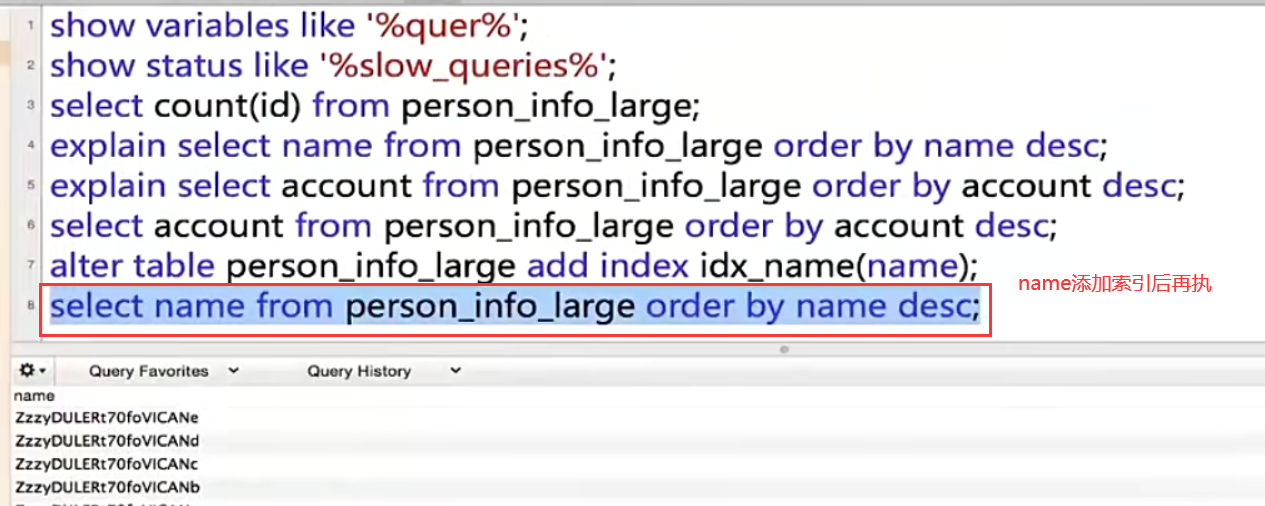结果发现，name添加索引之前，降序查询name是花费6.337729s，添加索引之后，降序查询name花费了3.479827s，原因就是B+树的结果集已经是有序的了。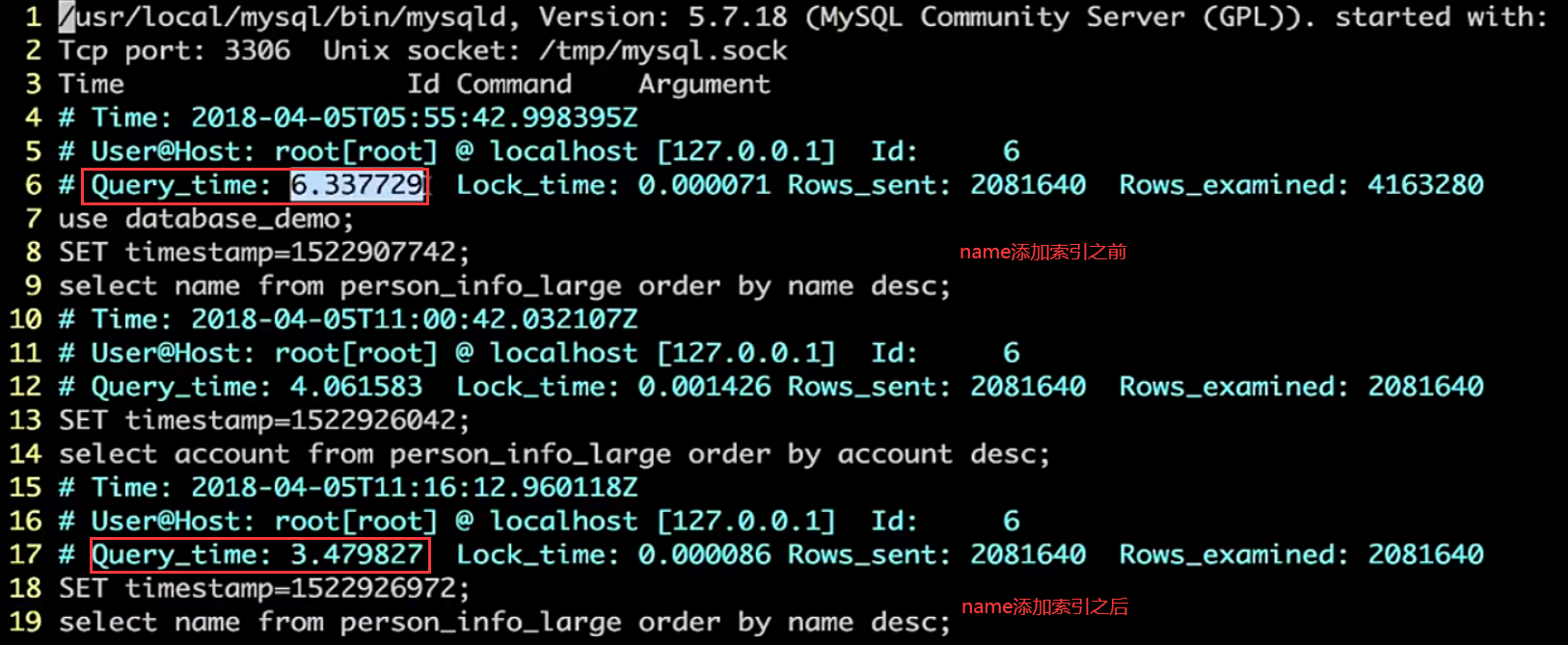5.当主键索引、唯一索引、普通索引都存在，查询优化器如何选择？
查询一下数据的条数，这里count(id)，分析一下sql执行计划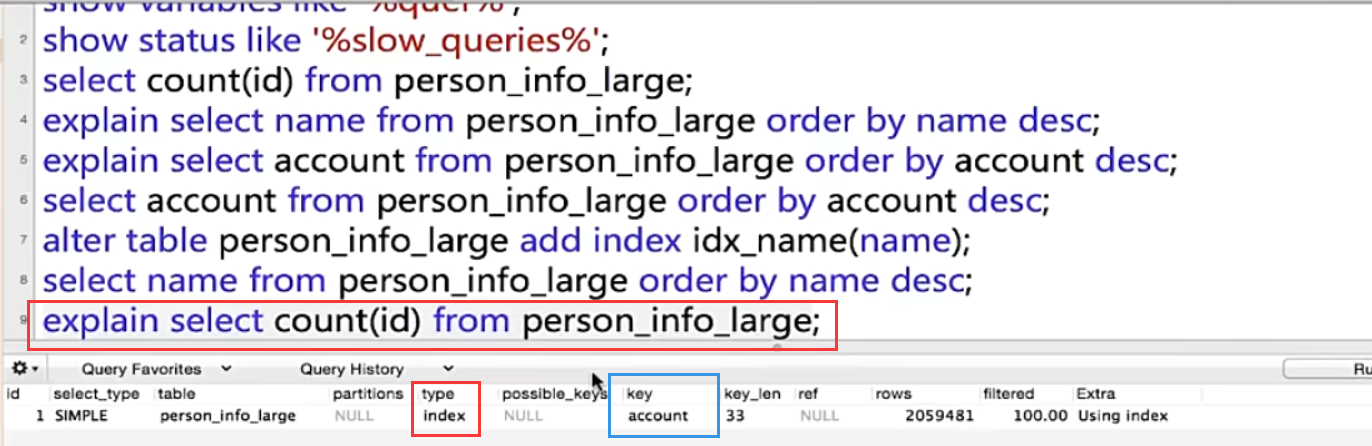这里实际使用的索引是account唯一索引。
分析一下：实际使用哪个索引是查询优化器决定的，B+树的叶子结点就是链表结构，遍历链表就可以统计数量，但是这张表，有主键索引、唯一索引、普通索引，优化器选择了account这个唯一索引，这肯定不会使用主键索引，因为主键索引是聚集索引，每个叶子包含具体的一个行记录（很多列的数据都在里面），而非聚集索引每个叶子只包含下一个主键索引的指针，很显然叶子结点包含的数据是越少越好，查询优化器就不会选择主键索引
当然，也可以强制使用主键索引，然后分析sql执行计划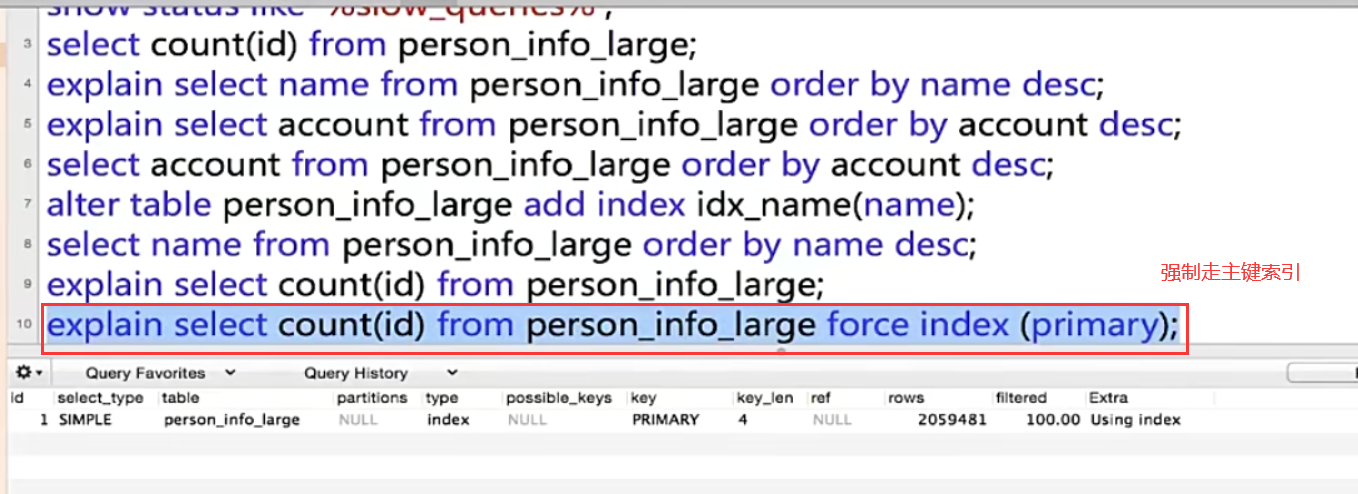我们看一下优化器默认使用唯一索引大致执行时间676ms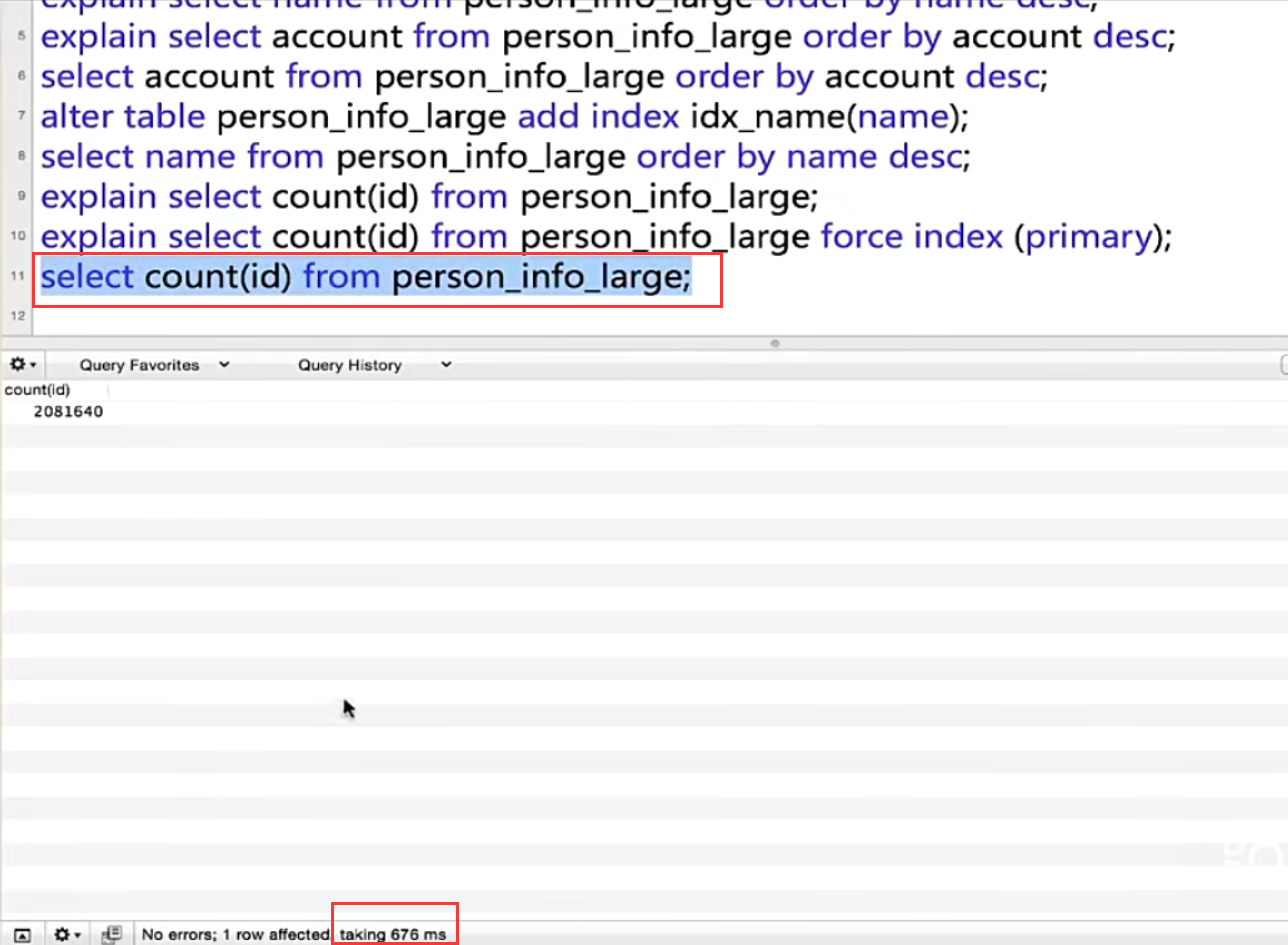强制使用主键索引大致执行时间779ms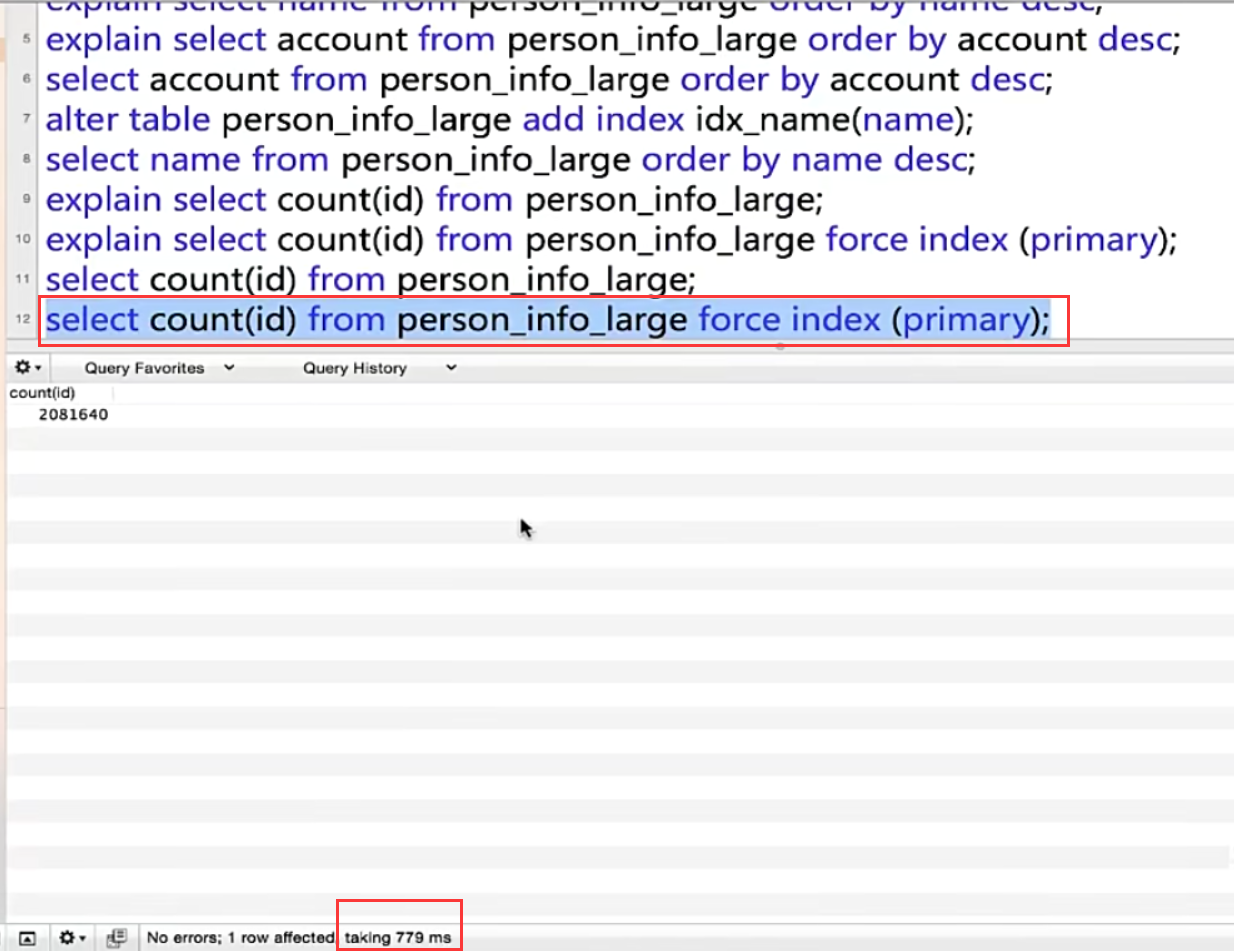我们可以用force index强制指定索引，然后去分析执行计划看看哪个索引是更好的，因为查询优化器选择索引不一定是百分百准确的，具体情况可以根据实际场景分析来确定是否使用查询优化器选择的索引。
欢迎一键三连~

有问题请留言，大家一起探讨学习

----------------------Talk is cheap, show me the code-----------------------
展开全文mysql慢查询 explain sql执行计划分析
• XX轮胎优化流程分析总结以持续提高组织业绩为目的，只为给你最适合、最想要XX轮胎优化流程分析总结参考，...该文档为XX轮胎优化流程分析总结，是一份很不错的参考资料，具有较高参考价值，感兴趣的可以下载看看
• 这一款优化流程原则(PEP)专为客户需求而设计，随着内外环境变化而变化，欢迎大家下载优化流程原则(PEP)参...该文档为优化流程原则(PEP)，是一份很不错的参考资料，具有较高参考价值，感兴趣的可以下载看看
• 优化大师 强大的系统优化程序，能够管理系统资源，系统内存，优化开机速度。
• 4—TD-SCDMA无线网络优化流程(含优化案例).pptx
• 高斯过程全局优化程序，使用高斯算法进行刀具加工的全局优化预测与拟合的matlab程序
• Matlab最优化程序，用信任域方法解决最优化问题。求出函数最小值。
• 著名优化程序NPSOL 使用SQP算法优化，里面含有NPSOL使用示例以及使用手册，主要可以用于解决非线性优化问题，本次上传的优化程序需要在安装编译器Fortran的环境下使用。解决非线性问题的规模还可以
• 网络优化流程和故障分析 上海大唐移动通信设备有限公司 2005 年 6 月 网络优化流程概述 ? 网络优化的工具 ? 网络优化工作步骤 ? 常见网络故障分析 目 录 网络优化工程的目的 提高平均的网络服务质量 尽可能地减少...
• WCDMA整网优化流程 目录 1 概述 5 1.1 全网优化定义 5 1.2 全网优化流程 5 2 单站优化 6 2.1 单站优化的目的 6 2.2 单站优化的测试内容和方法 6 2.2.1 基站基础数据库检查 6 2.2.2 站点配置验证 7 2.2.3 基站导频...
• 基于粒子群算法的配电网无功优化程序，利用了IEEE30节点进行MATLAB编程
• 尾递归优化流程解析 function tco(f) { var value; var active = false; var accumulated = []; return function accumulator() { accumulated.push(arguments); if (!active) { active = true; ...
尾递归优化流程解析
function tco(f) {
var value;
var active = false;
var accumulated = [];

return function accumulator() {
accumulated.push(arguments);
if (!active) {

active = true;
while (accumulated.length) {
// 用apply单纯是为了传入类数组，不用拆分
//等同于 var shifted = accumulated.shift();  value = f(...shifted);
value = f.apply(this, accumulated.shift())
//让顶层对象运用传进来的sum 方法，参数为，value一直到最后输出之前都是undefined
}

active = false;
return value;
}
};
}

var sum = tco(function(x, y) {
if (y > 0) {
return sum(x + 1, y - 1)
}
else {
return x
}
});
//tco返回一个accumulator函数,并通过这种方式将f传给accumulator

console.log(sum(1, 100)) //sum(1,100)等于运行了具有外部参数的accumulator(1,100)。参数是传给accumulator的。
// 101

具体流程解析
sum获取一个返回的函数accumulator
var sum = tco(function(x, y) {
if (y > 0) {
return sum(x + 1, y - 1)
}
else {
console.log('shit')
return x
}
});

这一步tco（function）返回一个函数accumulator(),具有外部变量value,active,accumulated。 因为accumulator中有使用，所以这些外部变量没有销毁。
sum(1,100) 即调用accumulator(1,100)  向数组accumulated中push入arguments对象
accumulated.push(arguments);

此时active为false，进入if语句  active转为true， 然后进入while循环。 这一步很重要，之后每一次调用accumulator函数只会向accumulated数组中传参。而不会进入if语句， 而只有第一次while循环反复调用sum(x+1,y-1)到结束。
即进入这样一个循环 ：

while (accumulated.length) {

value = f.apply(this, accumulated.shift())

sum(x+1,y-1) //即 accumulator(x+1,y-1)

accumulated.push(arguments)

}


一直到y=0时，sum(101，0)返回 101 ，不再向数组accumulated中传入数组，即离开循环。return 101 。
展开全文尾递归 蹦床函数
•cdma
• ## Sigmund的99行Matlab拓扑优化程序简析

万次阅读 多人点赞 2018-05-29 12:34:42
引言 Sigmund在2001年在Structural and Multidisciplinary Optimization 上...该论文后附带了一个Matlab拓扑优化程序。这个只有99行代码程序基于Matlab环境构建了一个完整的拓扑优化流程，其中包含：前处理(构建...
引言
Sigmund在2001年在Structural and Multidisciplinary Optimization 发表一篇名为 “A 99 line topology optimization code written in Matlab”论文。该论文附带一个使用Matlab编写的拓扑优化程序。这个只有99行代码的程序基于Matlab环境构建了一个完整的拓扑优化流程：前处理(构建有限元仿真模型), 有限元模型分析计算,拓扑优化迭代和后处理(分析结果显示)。Sigmund在论文中对这个程序的拓扑优化流程做了详细解释。本文仅从程序设计角度解析这段代码。
Sigmund的99行Matlab拓扑优化程序使用模块化方法设计，主要包含以下几个模块：  - 程序主流程  - 有限元模型求解模块  - Filter模块  - 单元刚度阵计算模块：计算平面四边形单元的刚度矩阵  - 优化模块： 使用优化准则法更新设计变量  - 目标函数计算和灵敏度分析模块  下面几个小节首先列出Sigmund99行拓扑优化程序完整代码，接着解释程序中5个全局变量在优化流程中的功用，然后对程序的各个功能模块做详细解析，最后总结程序的局限性并列出扩展程序功能时所需要修改的相应模块。
99行拓扑优化代码
Sigmund的99行Matlab拓扑优化程序如下所示
% a 99 line topology optimization code by Ole Sigmund,October 1999
clear
nelx=60;
nely=40;
volfrac=0.5;
penal=3.;
rmin=1.5;
% initialize
x(1:nely,1:nelx)=volfrac;
loop=0;
change=1;
% start ineration
while change>0.01
loop=loop+1;
xold=x;
% FE analysis
[U]=FE(nelx,nely,x,penal);
% objective function and sensitivity analysis
[KE]=lk;;
c=0.;
for ely=1:nely
for elx=1:nelx
n1=(nely+1)*(elx-1)+ely;
n2=(nely+1)*elx    +ely;
Ue=U([2*n1-1;2*n1;2*n2-1;2*n2;2*n2+1;2*n2+2;2*n1+1;2*n1+2],1);
c=c+x(ely,elx)^penal*Ue'*KE*Ue;
dc(ely,elx)=-penal*x(ely,elx)^(penal-1)*Ue'*KE*Ue;
end
end
% filtering of sensitivities
[dc]=check(nelx,nely,rmin,x,dc);
[x]=oc(nelx,nely,x,volfrac,dc);
% print result
change=max(max(x-xold))
disp(['It.:' sprintf( '%4i',loop) '    Obj.:' sprintf('   %10.4f',c) ...
'   Vol.:' sprintf('%6.3f',sum(sum(x))/(nelx*nely)) ...
'   ch.:' sprintf('%6.3f',change)])
% plot densities
colormap(gray);imagesc(-x);axis equal;axis tight; axis off;pause(1e-6);
end

% FE analysis
function [U]=FE(nelx,nely,x,penal)
[KE]=lk;
K=sparse(2*(nelx+1)*(nely+1),2*(nelx+1)*(nely+1));
F=sparse(2*(nely+1)*(nelx+1),1);
U=sparse(2*(nely+1)*(nelx+1),1);
for elx=1:nelx
for ely=1:nely
n1=(nely+1)*(elx-1)+ely;
n2=(nely+1)*elx    +ely;
edof=[2*n1-1;2*n1;2*n2-1;2*n2;2*n2+1;2*n2+2;2*n1+1;2*n1+2];
K(edof,edof)=K(edof,edof)+x(ely,elx)^penal*KE;
end
end
ip=(nelx+1)*(nely+1);
F(2*ip,1)=-1;

fixeddofs =[1:2*(nely+1)];
alldofs   =[1:2*(nely+1)*(nelx+1)];
freedofs  =setdiff(alldofs,fixeddofs);
% solving
U(freedofs,:)=K(freedofs,freedofs)\F(freedofs,:);
U(fixeddofs,:)=0;

% mesh-independency filter
function [dcn]=check(nelx,nely,rmin,x,dc)
dcn=zeros(nely,nelx);
for i=1:nelx
for j=1:nely
sum=0.0;
for k=max(i-floor(rmin),1):min(i+floor(rmin),nelx)
for l=max(j-floor(rmin),1):min(j+floor(rmin),nely)
fac=rmin-sqrt((i-k)^2+(j-l)^2);
sum=sum+max(0,fac);
dcn(j,i)=dcn(j,i)+max(0,fac)*x(l,k)*dc(l,k);
end
end
dcn(j,i)=dcn(j,i)/(x(j,i)*sum);
end
end

% Element stiffness matrix
function [KE]=lk
E=1.;
nu=0.3;
k=[1/2-nu/6 1/8+nu/8 -1/4-nu/12 -1/8+3*nu/8 ...
-1/4+nu/12 -1/8-nu/8 nu/6   1/8-3*nu/8];
KE=E/(1-nu^2)*[ k(1)  k(2)  k(3)  k(4)  k(5)  k(6)  k(7)  k(8)
k(2)  k(1)  k(8)  k(7)  k(6)  k(5)  k(4)  k(3)
k(3)  k(8)  k(1)  k(6)  k(7)  k(4)  k(5)  k(2)
k(4)  k(7)  k(6)  k(1)  k(8)  k(3)  k(2)  k(5)
k(5)  k(6)  k(7)  k(8)  k(1)  k(2)  k(3)  k(4)
k(6)  k(5)  k(4)  k(3)  k(2)  k(1)  k(8)  k(7)
k(7)  k(4)  k(5)  k(2)  k(3)  k(8)  k(1)  k(6)
k(8)  k(3)  k(2)  k(5)  k(4)  k(7)  k(6)  k(1)];

% optimality criteria update
function [xnew]=oc(nelx,nely,x,volfrac,dc)
l1=0;
l2=100000;
move=0.2;
while (l2-l1>1e-4)
lmid=0.5*(l2+l1);
xnew =max(0.001,max(x-move,min(1.,min(x+move,x.*sqrt(-dc./lmid)))));
if sum(sum(xnew))-volfrac*nelx*nely>0;
l1=lmid;
else
l2=lmid;
end
end             
变量初始化
nelx=60;   % x轴方向单元数量
nely=40;   % y轴方向单元数量
volfrac=0.5;  % 单元的材料体积比
penal=3.;  %  惩罚因子，将设计变量从[0,1]转化为指数函数，
%  主要目的是使单元材料密度更加"黑白分明",
%  惩罚因子就是这个指数函数的指数, 其值越大，效果越明显
rmin=1.5;  % 过滤半径，目的是预防出现棋盘格现象 
设计变量初始化
x(1:nely,1:nelx)=volfrac;  % 所有设计变量的赋初始值 
主程序
主程序迭代流程
% start ineration
while change>0.01
loop=loop+1;  % loop是迭代计数
xold=x;       % 将设计变量保存在 变量xold中
% FE analysis
[U]=FE(nelx,nely,x,penal);  % 有限元模型分析，将计算得到的各个节点位移值保存在数组U中
% objective function and sensitivity analysis
[KE]=lk;   % 计算单元刚度阵
c=0.;      % 保存目标函数(柔度)的变量，
%  遍历所有单元
for ely=1:nely
for elx=1:nelx
% 根据单元在设计域中位置 计算单元节点编号
n1=(nely+1)*(elx-1)+ely;  % 左上角节点编号
n2=(nely+1)*elx    +ely;  % 右上角节点编号
% 根据以上两个节点的编号 推演出 单元所有节点的自由度编号
% 这就限制了此程序只能使用四边形单元剖分四边设计域所得到的有限元模型
%
% 从数组 U 中根据节点自由度编号 提取该单元的 位移向量
Ue=U([2*n1-1;2*n1;2*n2-1;2*n2;2*n2+1;2*n2+2;2*n1+1;2*n1+2],1);
%  计算单元柔度，叠加到目标函数变量中
%  使用SIMP(Solid Isotropic Material with Penalization)材料插值模型
c=c+x(ely,elx)^penal*Ue'*KE*Ue;
%  计算灵敏度
dc(ely,elx)=-penal*x(ely,elx)^(penal-1)*Ue'*KE*Ue;
end
end
% filtering of sensitivities
[dc]=check(nelx,nely,rmin,x,dc);
[x]=oc(nelx,nely,x,volfrac,dc);
% print result
change=max(max(x-xold))
disp(['It.:' sprintf( '%4i',loop) '    Obj.:' sprintf('   %10.4f',c) ...
'   Vol.:' sprintf('%6.3f',sum(sum(x))/(nelx*nely)) ...
'   ch.:' sprintf('%6.3f',change)])
% plot densities
colormap(gray);imagesc(-x);axis equal;axis tight; axis off;pause(1e-6);
end 
优化模型为：

⎧⎩⎨⎪⎪⎪⎪⎪⎪⎪⎪⎪⎪⎪⎪⎪⎪mins.t.c(x)VρVKUxmin===<UTKU=∑ne=1(xe)puTeKeuefFx<1

{

m

i

n

c

(

x

)

=

U

T

K

U

=

∑

e

=

1

n

(

x

e

)

p

u

e

T

K

e

u

e

s

.

t

.

V

ρ

V

=

f

K

U

=

F

x

m

i

n

<

x

<

1

\left\{
\begin{array}{c c c l }
min & c(x) & = & U^{T}KU = \sum_{e = 1}^{n} (x_{e})^{p} u_{e}^{T}K_{e} u_{e}   \\
s.t. & & \\
& \frac{V_{\rho}}{V} & = & f  \\
& KU & = & F  \\
& x_{min} & < & x < 1        \\
\end{array}
\right.

代码 x(ely,elx)^penal*Ue’*KE*Ue 就是计算

(xe)puTeKeue

(

x

e

)

p

u

e

T

K

e

u

e

$(x_{e})^{p} u_{e}^{T}K_{e} u_{e}$  对

xe

x

e

$x_{e}$ 的梯度是

dcdx=−p(xe)p−1uTeKeue

d

c

d

x

=

−

p

(

x

e

)

p

−

1

u

e

T

K

e

u

e

\frac{dc}{dx} = - p (x_{e})^{p-1} u_{e}^{T}K_{e} u_{e}

此程序使用的SIMP(Solid Isotropic Material with Penalization)材料插值模型，具体代码为:
x(ely,elx)^penal ;

网格划分为
1-------(nely+1)+1------------------------------------nely+1)*nelx
|  (1,1)  |
2-------(nely+1)+2
|
...     -----------(nely+1)*(elx-1)+ely----(nely+1)*elx+ely
|                            |       (elx,ely)      |
...
|
nely+1---(nely+1)*2----------
单元节点编号顺序为：
         Node_1             Node_2
(nely+1)*(elx-1)+ely----(nely+1)*elx+ely
|       (elx,ely)      |
(nely+1)*(elx-1)+ely+1----(nely+1)*elx+ely+1
Node_4                Node_3        
过滤器模块
%%%%%%%%%% MESH-INDEPENDENCY FILTER %%%%%%%%%%%%%%%%%%%
function [dcn]=check(nelx,nely,rmin,x,dc)
% dcn 清零，dcn 用来保存 更新的 目标函数灵敏度
dcn=zeros(nely,nelx);
% 遍历所有单元
for i = 1:nelx
for j = 1:nely
sum=0.0;
% 遍历于这个单元相邻的单元
for k = max(i-floor(rmin),1):min(i+floor(rmin),nelx)
for l = max(j-floor(rmin),1):min(j+floor(rmin),nely)

%  sqrt((i-k)^2+(j-l)^2) 是计算此单元与相邻单元的距离
fac = rmin-sqrt((i-k)^2+(j-l)^2);
sum = sum + max(0,fac);

dcn(j,i) = dcn(j,i) + max(0,fac)*x(l,k)*dc(l,k);
end
end

dcn(j,i) = dcn(j,i)/(x(j,i)*sum);
end
end
Sigmund的论文中对此函数有详细解释，论文中符号与代码中变量名的对应关系如下表所示:
变量论文中符号rmin

rmin

r

m

i

n

$r_{min}$fac

Hf

H

f

$H_{f}$dc

cxe

c

x

e

$\frac{c}{x_{e}}$dcn

cxe¯

c

x

e

¯

$\bar { \frac{c}{x_{e}} }$
优化迭代模块
% optimality criteria update
function [xnew]=oc(nelx,nely,x,volfrac,dc)
l1=0;
l2=100000;
move=0.2;
while (l2-l1>1e-4)
lmid=0.5*(l2+l1);
xnew =max(0.001,max(x-move,min(1.,min(x+move,x.*sqrt(-dc./lmid)))));
if sum(sum(xnew))-volfrac*nelx*nely>0;
l1=lmid;
else
l2=lmid;
end
end             
这段代码就是实现下面这个方程式:

xnewe=⎧⎩⎨⎪⎪max(xmin,xe−m)xeBηemin(1,xe+m)ifififmax(xmin,xe−m)min(1,xe+m)≤≤xeBηe≤max(xmin,xe−m)xeBηe≤min(1,xe+m)xeBηe

x

e

n

e

w

=

{

m

a

x

(

x

m

i

n

,

x

e

−

m

)

i

f

x

e

B

e

η

≤

m

a

x

(

x

m

i

n

,

x

e

−

m

)

x

e

B

e

η

i

f

m

a

x

(

x

m

i

n

,

x

e

−

m

)

≤

x

e

B

e

η

≤

m

i

n

(

1

,

x

e

+

m

)

m

i

n

(

1

,

x

e

+

m

)

i

f

m

i

n

(

1

,

x

e

+

m

)

≤

x

e

B

e

η

x_{e}^{new} =
\left \{
\begin{array}{ c c c c l}
max(x_{min}, x_{e} - m) & if &                        &      & x_{e} B_{e}^{\eta} \leq max(x_{min}, x_{e}- m)  \\
x_{e} B_{e}^{\eta}      & if & max(x_{min}, x_{e}- m) & \leq & x_{e} B_{e}^{\eta} \leq min(1, x_{e}+m ) \\
min(1, x_{e}+m )        & if &   min(1, x_{e}+m )     & \leq & x_{e} B_{e}^{\eta} \\
\end{array}
\right  .

有限元模型求解模块
% FE analysis
function [U]=FE(nelx,nely,x,penal)
[KE]=lk; %计算单元刚度矩阵
K=sparse(2*(nelx+1)*(nely+1),2*(nelx+1)*(nely+1));
F=sparse(2*(nely+1)*(nelx+1),1);
U=sparse(2*(nely+1)*(nelx+1),1);
%组装整体刚度矩阵
for elx=1:nelx
for ely=1:nely
n1=(nely+1)*(elx-1)+ely;
n2=(nely+1)*elx    +ely;
edof=[2*n1-1;2*n1;2*n2-1;2*n2;2*n2+1;2*n2+2;2*n1+1;2*n1+2];
K(edof,edof)=K(edof,edof)+x(ely,elx)^penal*KE;
end
end
ip=(nelx+1)*(nely+1);
F(2*ip,1)=-1;  % 施加载荷

% 施加位移约束
fixeddofs =[1:2*(nely+1)];
alldofs   =[1:2*(nely+1)*(nelx+1)];
% 所有不受约束的节点自由度
freedofs  =setdiff(alldofs,fixeddofs);
% solving 求解线性方程组, 得到节点自由度的位移值
U(freedofs,:)=K(freedofs,freedofs)\F(freedofs,:);
U(fixeddofs,:)=0;  % 受约束的节点自由度的位移值 设为  0 
单元刚度阵模块
% Element stiffness matrix
function [KE]=lk
E=1.;
nu=0.3;
k=[1/2-nu/6 1/8+nu/8 -1/4-nu/12 -1/8+3*nu/8 ...
-1/4+nu/12 -1/8-nu/8 nu/6   1/8-3*nu/8];
KE=E/(1-nu^2)*[ k(1)  k(2)  k(3)  k(4)  k(5)  k(6)  k(7)  k(8)
k(2)  k(1)  k(8)  k(7)  k(6)  k(5)  k(4)  k(3)
k(3)  k(8)  k(1)  k(6)  k(7)  k(4)  k(5)  k(2)
k(4)  k(7)  k(6)  k(1)  k(8)  k(3)  k(2)  k(5)
k(5)  k(6)  k(7)  k(8)  k(1)  k(2)  k(3)  k(4)
k(6)  k(5)  k(4)  k(3)  k(2)  k(1)  k(8)  k(7)
k(7)  k(4)  k(5)  k(2)  k(3)  k(8)  k(1)  k(6)
k(8)  k(3)  k(2)  k(5)  k(4)  k(7)  k(6)  k(1)];
4节点矩形单元的4个节点在单元局部坐标系中坐标分别为：
Nodexy

(x1,y1)

(

x

1

,

y

1

)

$(x_{1}, y_{1})$00

(x2,y2)

(

x

2

,

y

2

)

$(x_{2}, y_{2})$10

(x3,y3)

(

x

3

,

y

3

)

$(x_{3}, y_{3})$11

(x4,y4)

(

x

4

,

y

4

)

$(x_{4}, y_{4})$01
各节点形函数设为

⎧⎩⎨⎪⎪⎪⎪⎪⎪⎪⎪N1N2N3N4====(x−1)(y−1)−x(y−1)xy−(x−1)y

{

N

1

=

(

x

−

1

)

(

y

−

1

)

N

2

=

−

x

(

y

−

1

)

N

3

=

x

y

N

4

=

−

(

x

−

1

)

y

\left \{
\begin{array}{c c c c c}
N_{1}  & =   &  (x - 1 ) (y - 1)  \\
N_{2}  & =   & - x (y - 1)  \\
N_{3}  & =  &  x y  \\
N_{4}  & =  &  -(x - 1 ) y  \\
\end{array}
\right .

以各节点形函数为基函数构建线性单元的位移场。单元内任一点

(x,y)

(

x

,

y

)

$(x,y)$ 处的位移可由四个节点的变形通过单元形函数求得：

{u(x,y)v(x,y)==∑4i=1Ni(x,y)ui∑4i=1Ni(x,y)vi

{

u

(

x

,

y

)

=

∑

i

=

1

4

N

i

(

x

,

y

)

u

i

v

(

x

,

y

)

=

∑

i

=

1

4

N

i

(

x

,

y

)

v

i

\left \{
\begin{array}{c c l}
u(x,y) & = & \sum_{i=1}^{4}N_{i}(x,y)u_{i} \\
v(x,y) & = & \sum_{i=1}^{4}N_{i}(x,y)v_{i} \\
\end{array}
\right  .

其中

ui,vi

u

i

,

v

i

$u_{i}, v_{i}$ 分别为各个节点在单元局部坐标系中

x

x

$x$ 坐标轴向和y$y$$y$ 坐标轴向的位移分量。
根据几何方程，应变为：

ξxx=∂u∂x=∂N1∂xu1+⋯+∂N4∂xu4

ξ

x

x

=

∂

u

∂

x

=

∂

N

1

∂

x

u

1

+

⋯

+

∂

N

4

∂

x

u

4

\xi_{xx} = \frac{\partial u }{\partial x} = \frac{\partial N_{1}}{\partial x} u_{1} + \cdots + \frac{\partial N_{4}}{\partial x} u_{4}\\

ξyy=∂v∂y=∂N1∂yv1+⋯+∂N4∂yv4

ξ

y

y

=

∂

v

∂

y

=

∂

N

1

∂

y

v

1

+

⋯

+

∂

N

4

∂

y

v

4

\xi_{yy} = \frac{\partial v}{\partial y} = \frac{\partial N_{1}}{\partial y} v_{1} + \cdots + \frac{\partial N_{4}}{\partial y} v_{4} \\

ξxy=∂v∂x+∂u∂y=∂N1∂yu1+∂N1∂xv1+⋯+∂N4∂yu4+∂N4∂xv4

ξ

x

y

=

∂

v

∂

x

+

∂

u

∂

y

=

∂

N

1

∂

y

u

1

+

∂

N

1

∂

x

v

1

+

⋯

+

∂

N

4

∂

y

u

4

+

∂

N

4

∂

x

v

4

\xi_{xy} = \frac{\partial v}{\partial x} + \frac{\partial u }{\partial y}
= \frac{\partial N_{1}}{\partial y} u_{1} + \frac{\partial N_{1}}{\partial x} v_{1} + \cdots
+ \frac{\partial N_{4}}{\partial y} u_{4} + \frac{\partial N_{4}}{\partial x} v_{4}

则单元B阵为

B=⎡⎣⎢⎢⎢⎢∂N1(x,y)∂x0∂N1(x,y)∂y⋯⋯⋯0∂N4(x,y)∂y∂N4(x,y)∂x⎤⎦⎥⎥⎥⎥

B

=

[

∂

N

1

(

x

,

y

)

∂

x

⋯

0

0

⋯

∂

N

4

(

x

,

y

)

∂

y

∂

N

1

(

x

,

y

)

∂

y

⋯

∂

N

4

(

x

,

y

)

∂

x

]

B =
\left [
\begin{array}{c c  c}
\frac{\partial N_{1}(x,y)}{\partial x} & \cdots & 0 \\
0  & \cdots & \frac{\partial N_{4}(x,y)}{\partial y}   \\
\frac{\partial N_{1}(x,y)}{\partial y}   & \cdots & \frac{\partial N_{4}(x,y)}{\partial x}  \\
\end{array}
\right ]

材料本构阵为：

D=E1−ν2⎡⎣⎢1ν0ν10001−ν2⎤⎦⎥

D

=

E

1

−

ν

2

[

1

ν

0

ν

1

0

0

0

1

−

ν

2

]

D
=
\frac{E}{1-\nu^{2}}
\left [
\begin{array}{c c c}
1  &  \nu  & 0 \\
\nu & 1 & 0  \\
0 &  0  & \frac{1-\nu}{2} \\
\end{array}
\right ]

单元刚度阵为：

K=∫BTDBds

K

=

∫

B

T

D

B

d

s

K = \int B^{T} D B ds

其中

K11=E1−ν2∫10∫10((y−1)2+1−ν2(x−1)2)dxdy=E1−ν2(12−ν6)

K

11

=

E

1

−

ν

2

∫

0

1

∫

0

1

(

(

y

−

1

)

2

+

1

−

ν

2

(

x

−

1

)

2

)

d

x

d

y

=

E

1

−

ν

2

(

1

2

−

ν

6

)

K_{11} =\frac{E}{1-\nu^{2}} \int_{0}^{1}\int_{0}^{1} ( (y-1)^{2} + \frac{1-\nu}{2}(x-1)^{2} )dxdy
= \frac{E}{1-\nu^{2}}( \frac{1}{2} - \frac{ \nu }{6})

其余的

Kij

K

i

j

$K_{ij}$依次类推。

程序的局限性
Sigmund的99行Matlab拓扑优化程序 主要关注于拓扑优化算法是验证. 以牺牲了程序的通用性为代价，短短的99行代码中完成一个完整的拓扑优化流程。整个程序代码简洁、计算高效，但各程序模块的功能能省就省，往往使用最简单最直接的方法实现，存在一定的局限性，例如：  - 设计域默认是矩形  - 只能处理一种单元– 平面四边形单元  - 只能使用一种各向同性材料  - Filter函数依赖于设计域网格划分方式
修改程序
如果要改变优化模型和有限元模型，调整项与相应的功能模块如下表所示：
调整项功能模块函数名优化模型主程序优化算法目标函数计算模块OC边界条件有限元模型求解模块FE材料类型单元刚度阵模块lk过滤算法过滤器模块check材料插值模型主程序
展开全文• 程序是199行水平集拓扑优化的经典程序，是香港中文大学王煜教授团队所写，内容为2D结构的刚度优化...